• 典型相关分析matlab源代码，直接运行即可，用于典型变化，图像处理中的多元变化检测等。 典型相关分析matlab源代码，直接运行即可，用于典型变化，图像处理中的多元变化检测等。
• MATLAB源码集锦-特征降维，特征融合，相关分析等多元数据分析的典型相关分析代码
• 典型相关分析matlab实现代码DCCA：深度规范相关分析 这是带有pytorch的Python中的深度规范相关性分析（DCCA或Deep CCA）的实现，它支持多GPU训练。 DCCA是CCA的非线性版本，它使用神经网络代替线性变换器作为映射...
• 典型相关分析MATLAB实现，注释详细 可以直接调用
• 为在特征融合中综合利用数据的类别信息和数据结构中所蕴含的自然鉴别信息, 提出一种基于局部稀疏表示和线性鉴别分析的典型相关分析算法. 首先利用局部稀疏表示模型, 以较小的计算复杂度获取局部稀疏重构矩阵; 然后在...
• 中实现典型相关分析 SPSS?11.0 15.1?典型相关分析 15.1.1?方法简介 在相关分析一章中我们主要研究的是两个变量间的相关顶多调整其他因素的作用而已 如果要研究一个变量和一组变量间的相关则可以使用多元线性回归方程...
• 对于两个变量，是用它们的相关系数来衡量它们之间的线性相关关系的。...对于这类问题的研究引进了典型相关系数的概念，从而找到了揭示两组变量之间线性相关关系的一种统计分析方法——典型相关分析
• 典型相关分析 在统计中，规范相关分析（CCA）是从互协方差矩阵推断信息的一种方式。 如果我们有两个随机变量的向量X =（X_1，...，X_n）和Y =（Y_1，...，Y_m），并且变量之间存在相关性，则典范相关分析将找到的...HTML
• 然后采用核典型相关分析（kernel canonical correlation analysis，KCCA）来提取独立分量与位置信息之间的典型相关特征；最后结合传统定位算法如加权K近邻法（weighted K nearest neighbors，WKNN）、支持向量机...无线局域网 室内定位 接收信号强度 独立成分分析
• 按类型分类的数学建模获奖论文，非常有用，可以系统的参考。
• 学习SPSS典型相关分析的好教程，利用spss进行典型相关分析
• ## 典型相关分析

千次阅读 2019-06-11 14:27:12
典型相关分析函数：[a,b,r,u,v,stats] = cononcorr(x,y): param: 　x：原始变量x矩阵，每列一个自变量指标，第i列是 xi 的样本值 　y：原始变量y矩阵，每列一个因变量指标，第j列是 yj 的样本值 return: 　a：...
典型相关分析函数：[a,b,r,u,v,stats] = cononcorr(x,y):
param:
x：原始变量x矩阵，每列一个自变量指标，第i列是 xi 的样本值
y：原始变量y矩阵，每列一个因变量指标，第j列是 yj 的样本值
return:
a：自变量x的典型相关变量系数矩阵，每列是一组系数。
列数为典型相关变量数
b：因变量y的典型相关变量系数矩阵，每列是一个系数
r： 典型相关系数。即第一对<u1,v1>之间的相关系数、第二对<u2,v2>之间的相关系数…
u：对于X的典型相关变量的值
v：对于Y的典型相关变量的值
stats：假设检验的值<详细用一下就知道了>

主成分分析（PCA）是把原始有相关性变量，线性组合出无关的变量（投影），以利用主成分变量进行更加有效的分析。 而典型相关分析（CCA）的思想是：
分析自变量组 X = [x1,x2,x3…xp]，因变量组 Y = [y1,y2,y3…yq] 之间的相关性。(注意这里X的每一个自变量x1是个列向量，代表有多个观测值)。
如果采用传统的相关分析，只要求X的每一个变量与Y的每一个变量的相关系数，从而组成相关系数矩阵 R = [rij]p*q ,rij表示第i个自变量xi与第j个因变量yj之间的相关系数。
然而，这是有缺陷的：只粗暴的考虑了X与Y的关系，却忽略了X自变量之间也可能有相关关系，Y因变量之间亦如此。
解决的方法类似于主成分分析，我们可以把X提取出主成分，Y也提取出主成分，从而X、Y内部线性不相关了，这样利用主成分研究X与Y之间相关性就解决了上述缺点。
1. 典型相关分析
典型相关分析：
假设
自变量组：X = [x1,x2,x3…xp]
因变量组：Y = [y1,y2,y3…yq]
注意，xi与yj都是相同维度的列向量。
要求
分析X与Y之间的相关性

2 直观描述
首先在X中找出线性组合u1, 在Y中找出线性组合v1，使得 r(u1,v1)达到最大。
其次，在X中找第二个线性组合u2，Y中找第二个线性组合v2,要求使得u2与u1线性不相关，v2与v1线性不相关，并且: r(u2,v2)达到次大。
继续。直到两组变量之间的相关性被提取完毕。
假设检验：
大体的意思：先进行假设，原假设‘不能进行典型相关分析’，然后检验，一直到所有的(U,V)对才可以结束，检验的方法：似然比统计量。
展开全文• 详细讲解如何用matlab的内置函数做线性分析，有例子，方法非常简单，对做线性分析的朋友很有用
• 数学建模分析——SAS程序——典型相关分析问题模板，这里以五年前后各指标相应的数据进行对比，分析得出了相关的结论，用以之后的进一步分析。
• 射频识别在SSVEP-BCI基于使用MULTISET典型相关分析研究论文
• CCA———典型相关分析，很经典的论文，需要的拿走，但是因为我也是菜鸟，不得已，还是得要点分。
• 文章目录一、引述1.概念2.示例说明 一、引述 ...典型相关分析用于研究两组变量（每组变量中都可能有多个指标）之间相关关系的一种多元统计方法。它能够揭示出两组变量之间的内在联系。 2.示例说明 ...


文章目录
一、引述1.概念2.何为两组变量呢？3. 本文主要内容
二、典型相关分析1. 基本思路2. 基本思想3. 基本思路
三、关键步骤（看不懂的话，可以先看四）四、使用SPSS进行典型相关分析1.导入数据2. 检验数据类型3. 对数据进行典型相关分析4.导出分析结果6.修改原文件中表格的名称
五、对结果进行分析1.分析典型相关系数表2. 分析标准化典型相关系数3. 分析典型载荷4. 分析已解释的方差比例
六、资料链接

一、引述
1.概念
典型相关分析用于研究两组变量（每组变量中都可能有多个指标）之间相关关系的一种多元统计方法。它能够揭示出两组变量之间的内在联系。在一元统计分析中，用相关系数来衡量两个随机变量之间的线性相关关系；用复相关系数研究一个随机变量和多个随机变量的线性相关关系。然而，这些统计方法在研究两组变量之间的相关关系时却无能为力。比如要研究生理指标与训练指标的关系，居民生活环境与健康状况的关系，人口统计变量（户主年龄、家庭年收入、户主受教育程度）与消费变量（每年去餐馆就餐的频率、每年出外看电影的频率）之间是否具有相关关系 ？阅读能力变量（阅读速度、阅读才能）与数学运算能力变量（数学运算速度、数学运算才能）**是否相关？这些多变量间的相关性如何分析？
2.何为两组变量呢？
下图是测量的20名学生的生理指标与训练指标。第一组是生理指标变量，有体重、腰围和脉搏；第二组是训练指标变量，有引体向上次数、起坐次数和跳跃次数。要求测量生理指标与训练指标这两组变量之间的关系。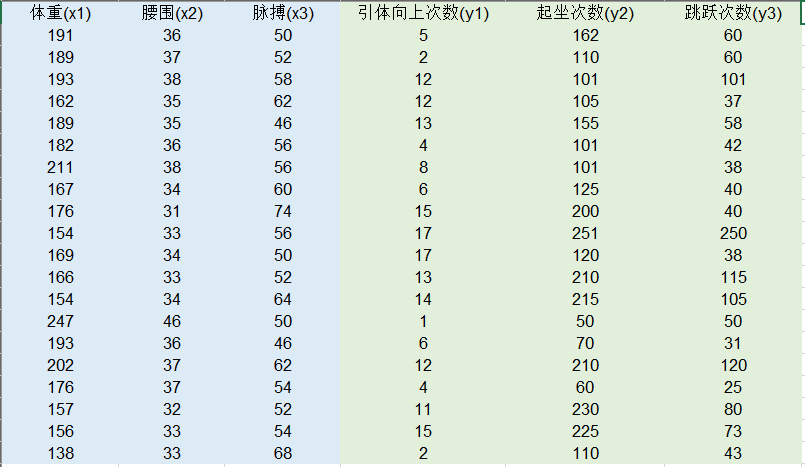在本题中，如果我们直接对这些变量（诸如体重、胸围等变量）的相关性进行两两分析，很难得到题干所要求的测量生理指标与训练指标这两组变量之间的关系。所以，我们引入一种新的分析方法：典型相关分析。
3. 本文主要内容
本文主要目的在于介绍典型相关分析的基本思想和解题步骤以及讲解如何使用SPSS24.0解决该类数学建模问题。如果要进行论文写作，我们需要掌握典型相关分析的原理及方法。这一部分，我将在后面的专栏中结合相关获奖论文进行说明。
二、典型相关分析
1. 基本思路
在上例中，我们可以采用这样的解决思路：由于两组变量中都含有多个变量指标，每组变量中定然会有代表性的变量。这样，找到代表性的变量，我们便可以把 多个变量与多个变量之间的相关变成两个具有代表性的变量之间的相关 。代表性变量：能较为综合、全面的衡量所在组的内在规律。一组变量最简单的综合形式就是该组变量的线性组合。
2. 基本思想
典型相关分析由Hotelling提出，其基本思想和主成分分析非常相似。
首先在每组变量中找出变量的线性组合，使得两组的线性组合之间具有最大的相关系数；然后选取和最初挑选的这对线性组合不相关的线性组合，使其配对，并选取相关系数最大的一对。如此继续下去，知道两组变量之间的相关性被提取完毕为止。被选出的线性组合配对称为典型变量，它们的相关系数称为典型相关系数。典型相关系数度量了这两组变量之间的强度。
3. 基本思路
一般情况下，假设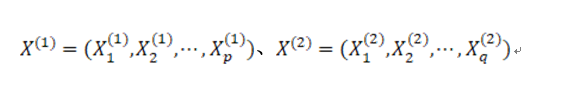是两个相互关联的随机变量，分别在两组变量中选取若干有代表性的综合变量Ui、Vi，使得每一个综合变量是原变量的线性组合，即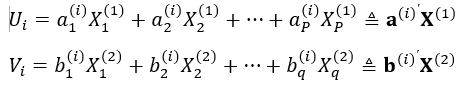当然，综合变量的组数是不确定的，如果第一组就能代表原样本数据大部分的信息，那么一组就足够了。如果第一组反映的信息不够，我们就需要找第二组数据。  为了让所找到的第二组数据的信息更加有效，我们需要保证第二组数据和第一组数据不相关，即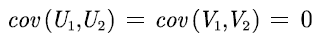对于数学的部分，我就不再过多阐述（无力.jpg）。感兴趣的同学可以自行查找资料。上面一点便是我们所要达到的终极目的。
三、关键步骤（看不懂的话，可以先看四）
假设我们所研究的两组数据服从联合正态分布。对这两组变量的相关性进行检验（构造似然比统计量）。
H0：两组变量的协差阵为0（两组变量无关）；H1：两组变量的协差阵不为0（两组变量有关）用于检验的似然比统计量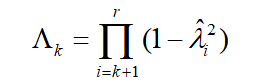p值小于0.5（0.1）表示在95%（90%）的置信水平下拒绝原假设， 即认为两组变量有关。 确定典型相关变量的个数（直接看典型相关系数对应的p值即可）利用标准化后的典型相关变量分析问题 为了消除量纲和数量级别的影响，必须对数据先做标准化变换处理，然后再做典型相关分析。进行典型载荷分析计算前r个典型变量对样本总方差的贡献
四、使用SPSS进行典型相关分析
1.导入数据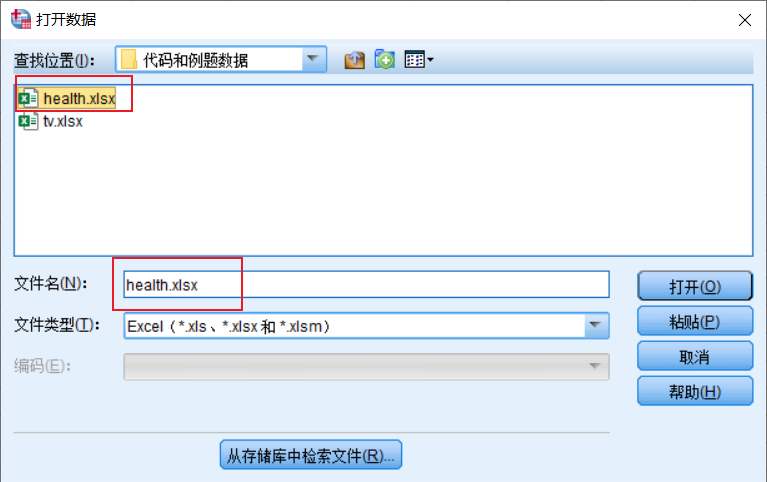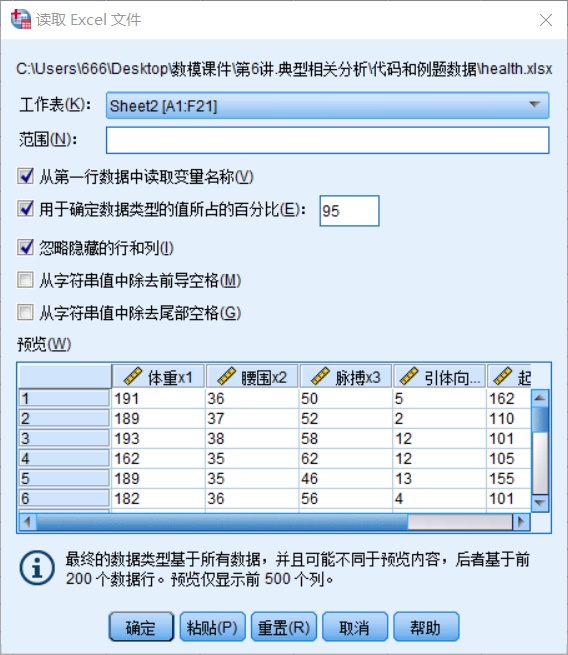2. 检验数据类型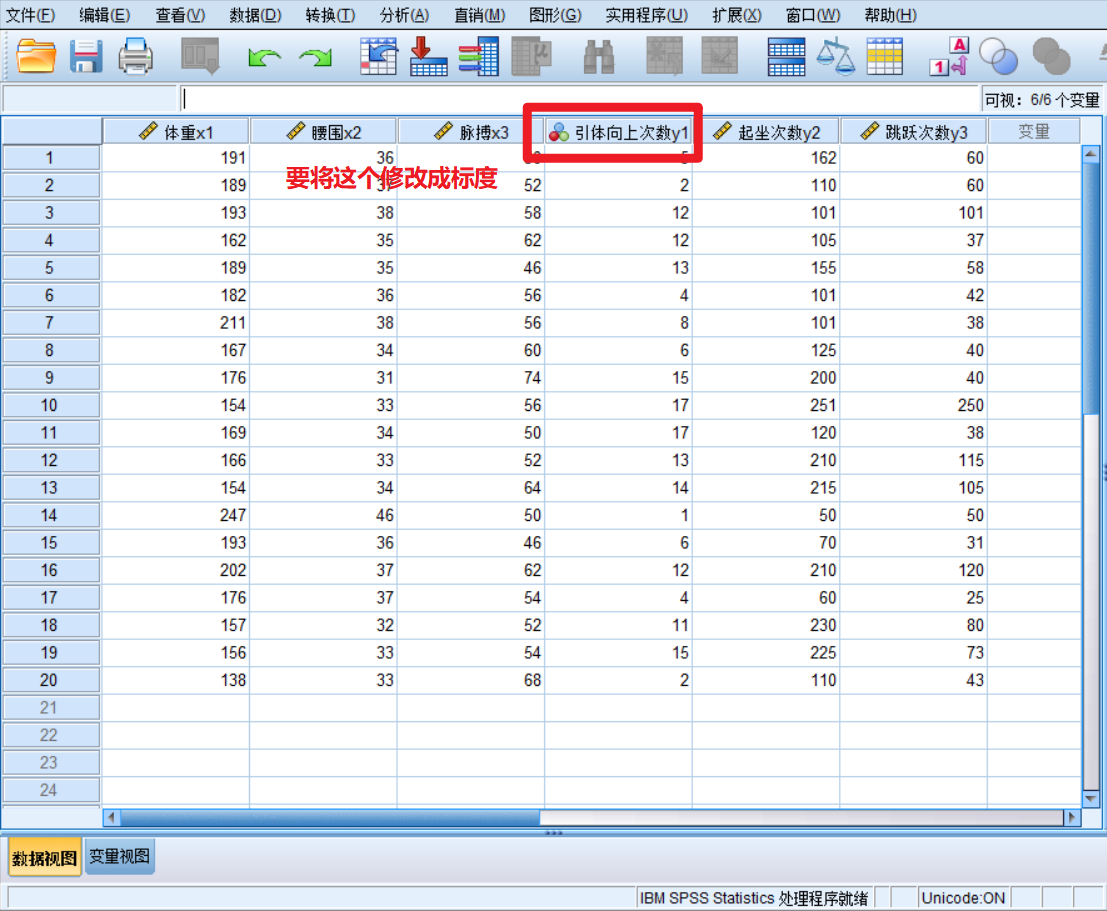点击左下角的变量视图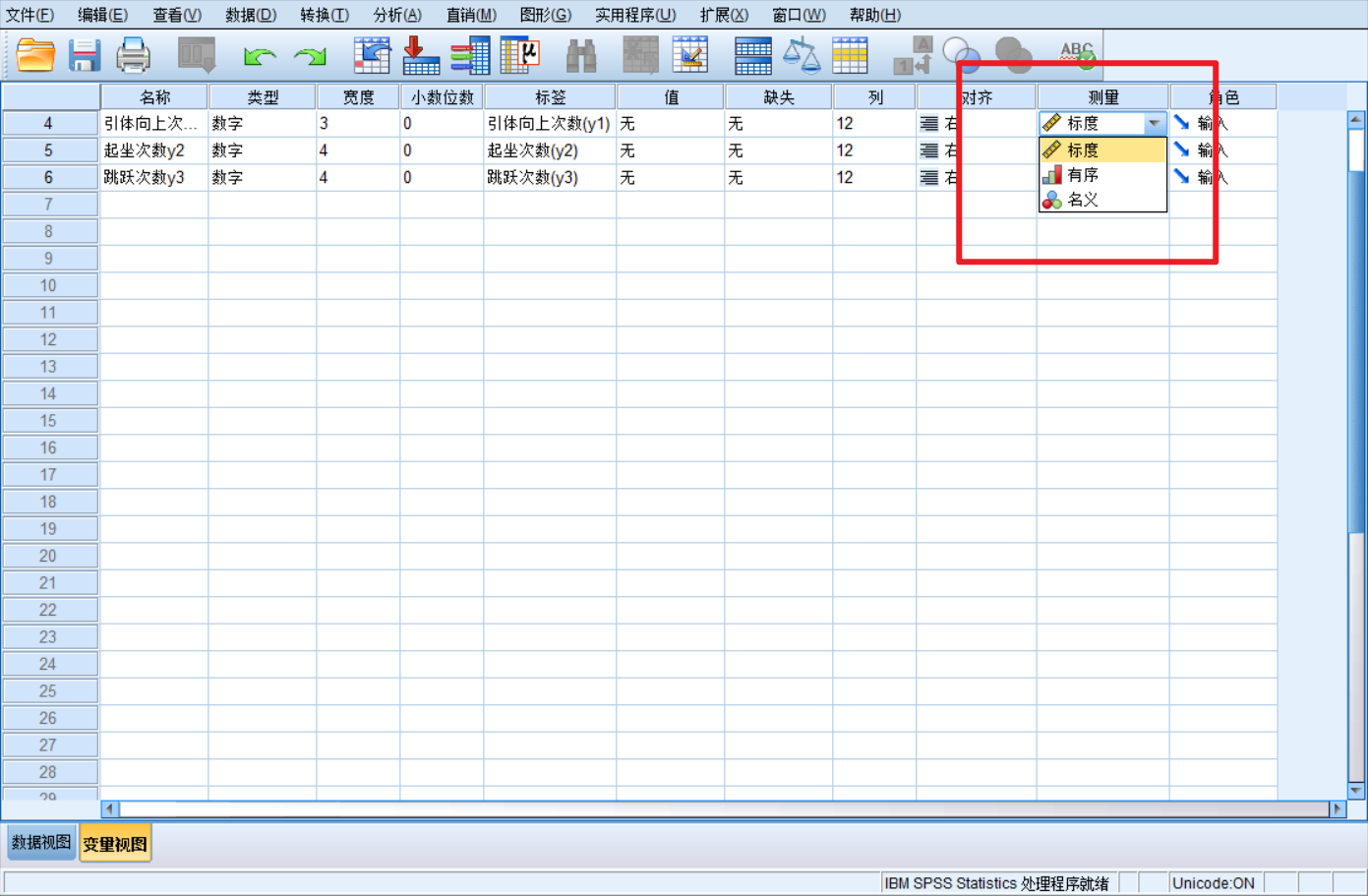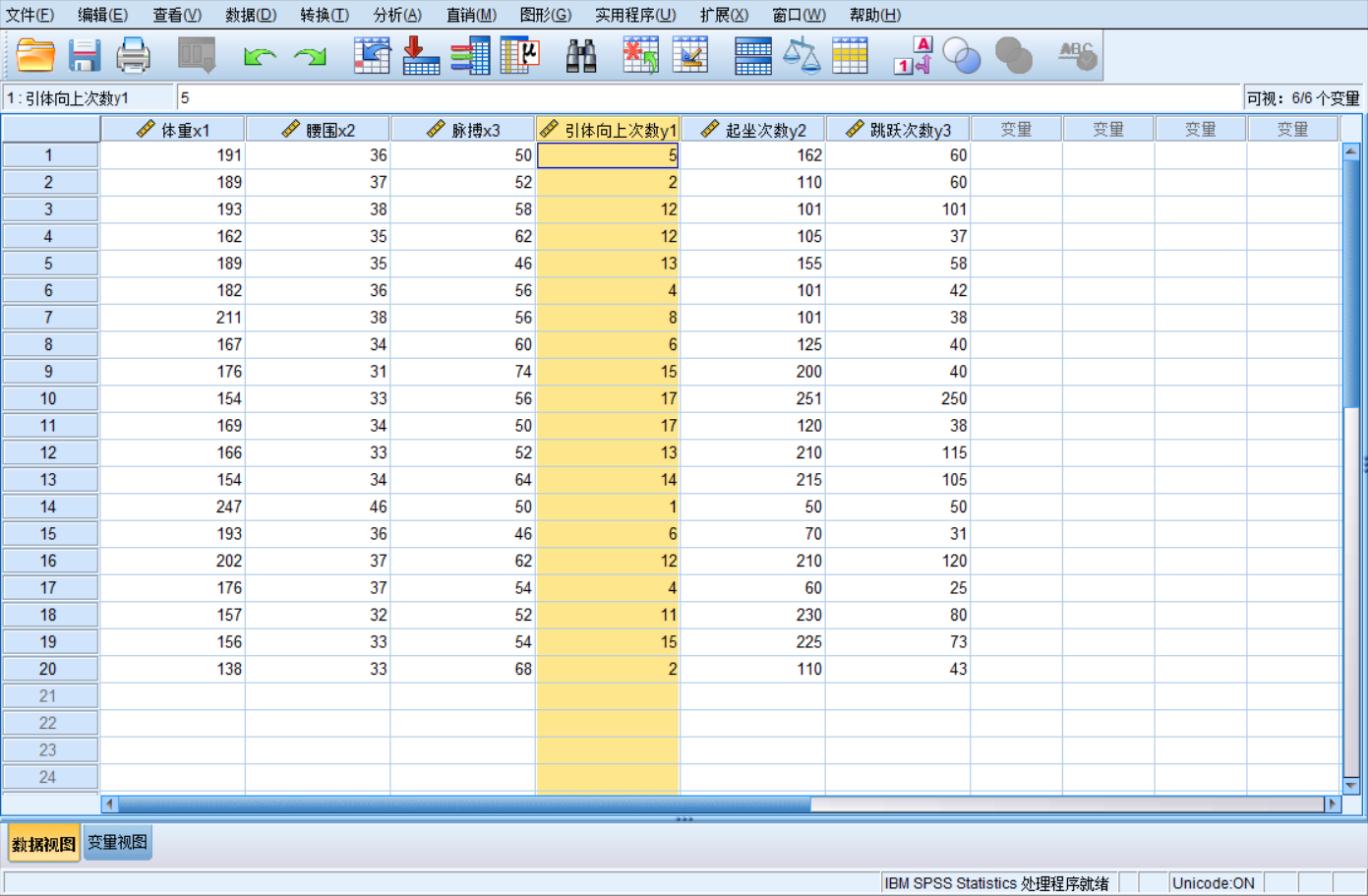3. 对数据进行典型相关分析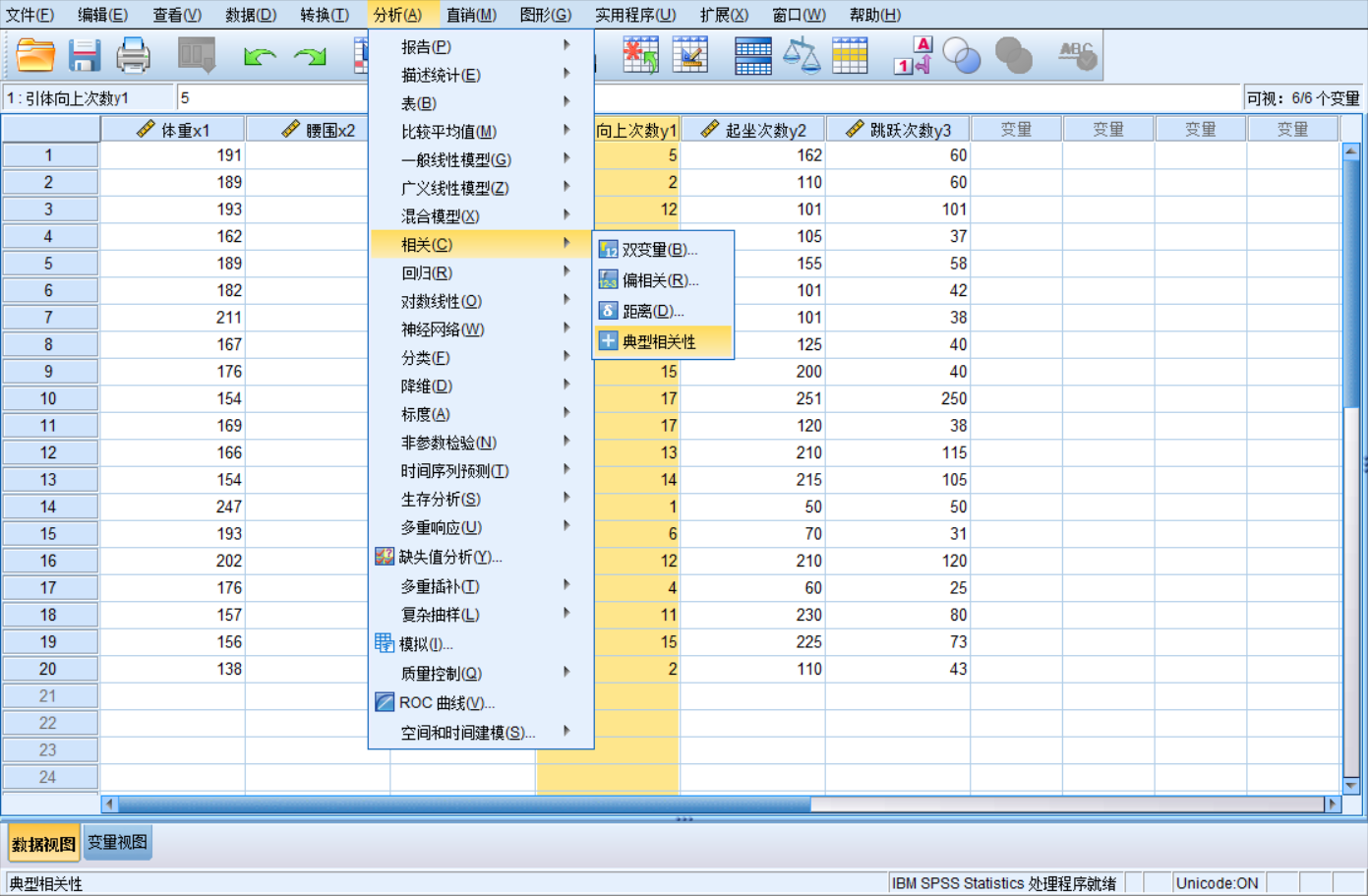按照题干要求将变量进行分组（按住ctrl，可以进行多个选中）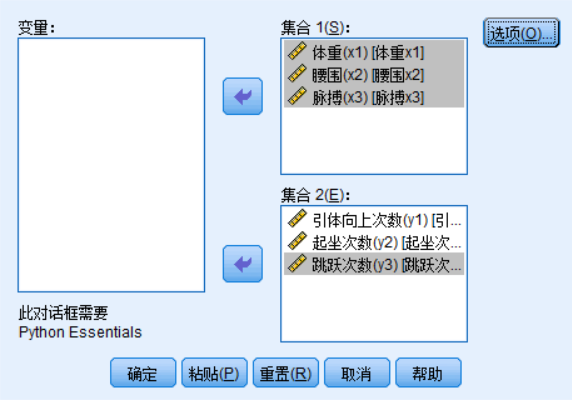之后便得到如下内容：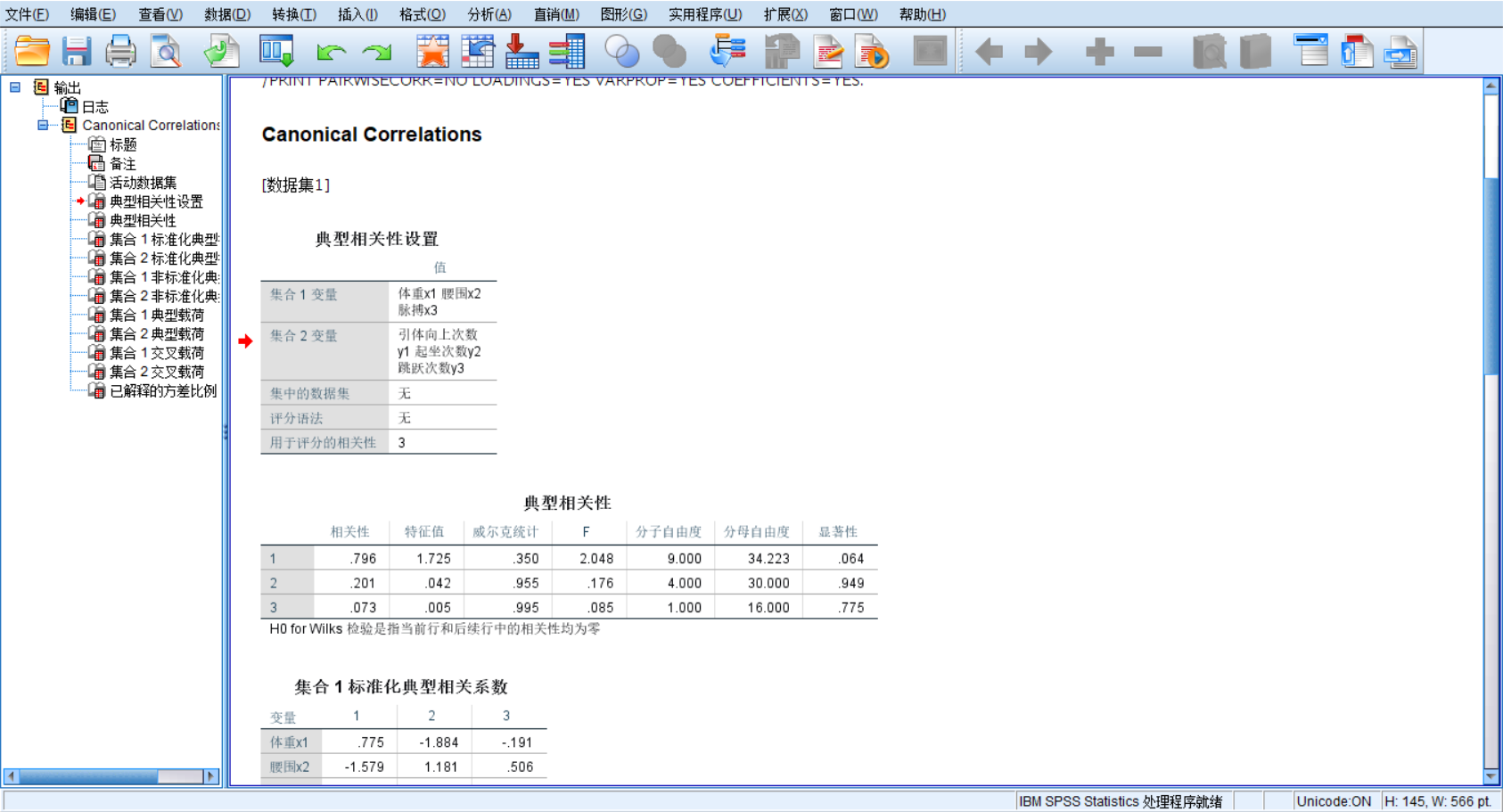4.导出分析结果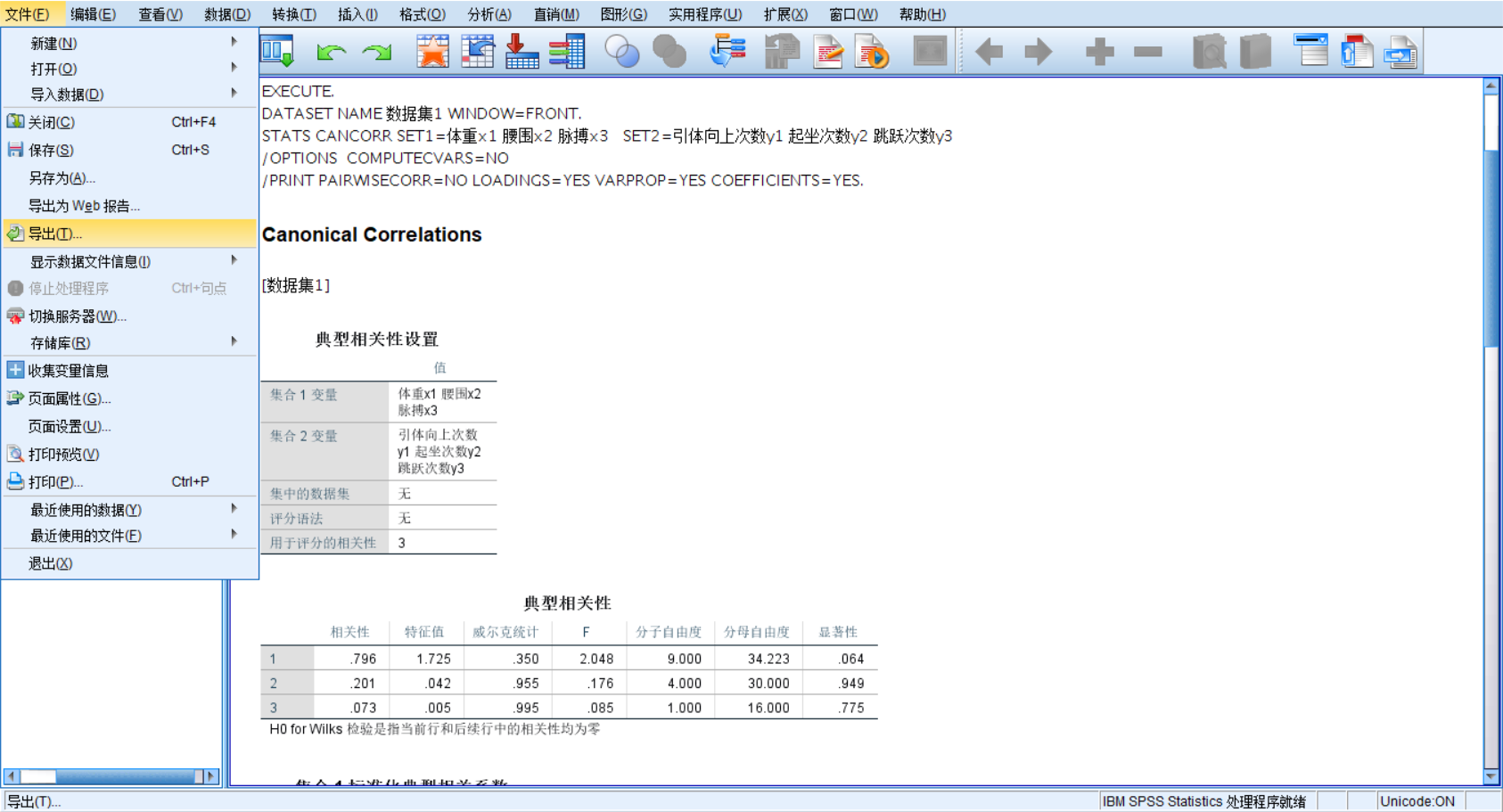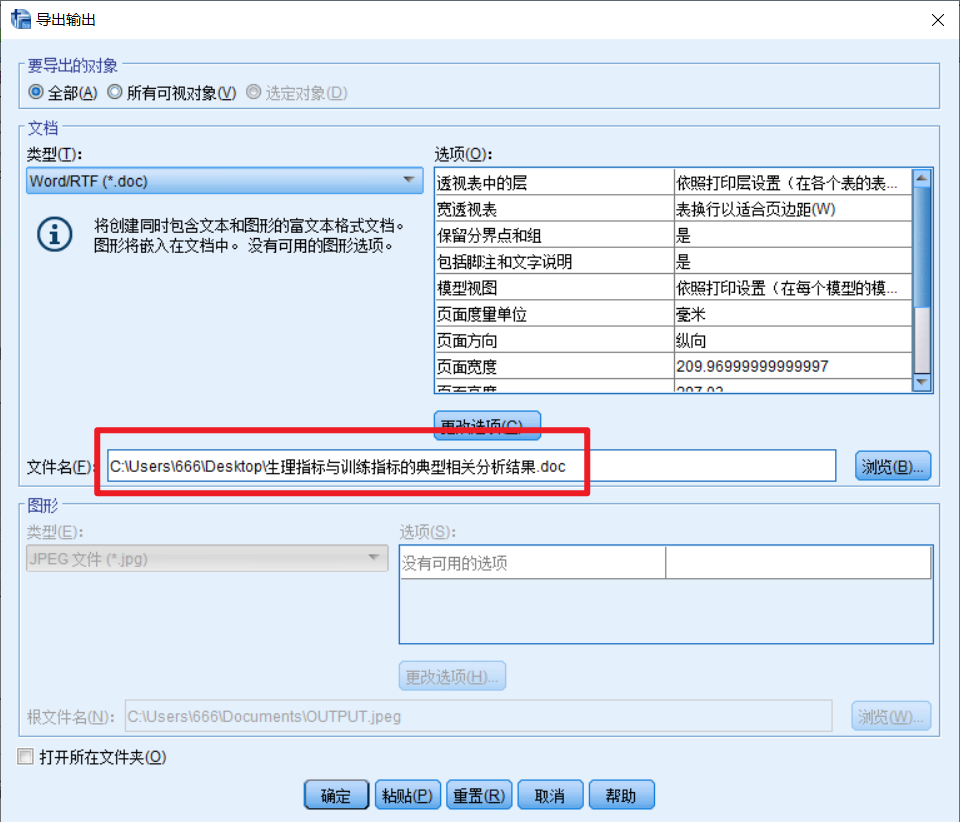于是我们便在桌面上得到了该文件。6.修改原文件中表格的名称
下面是刚打开的原文件表格名称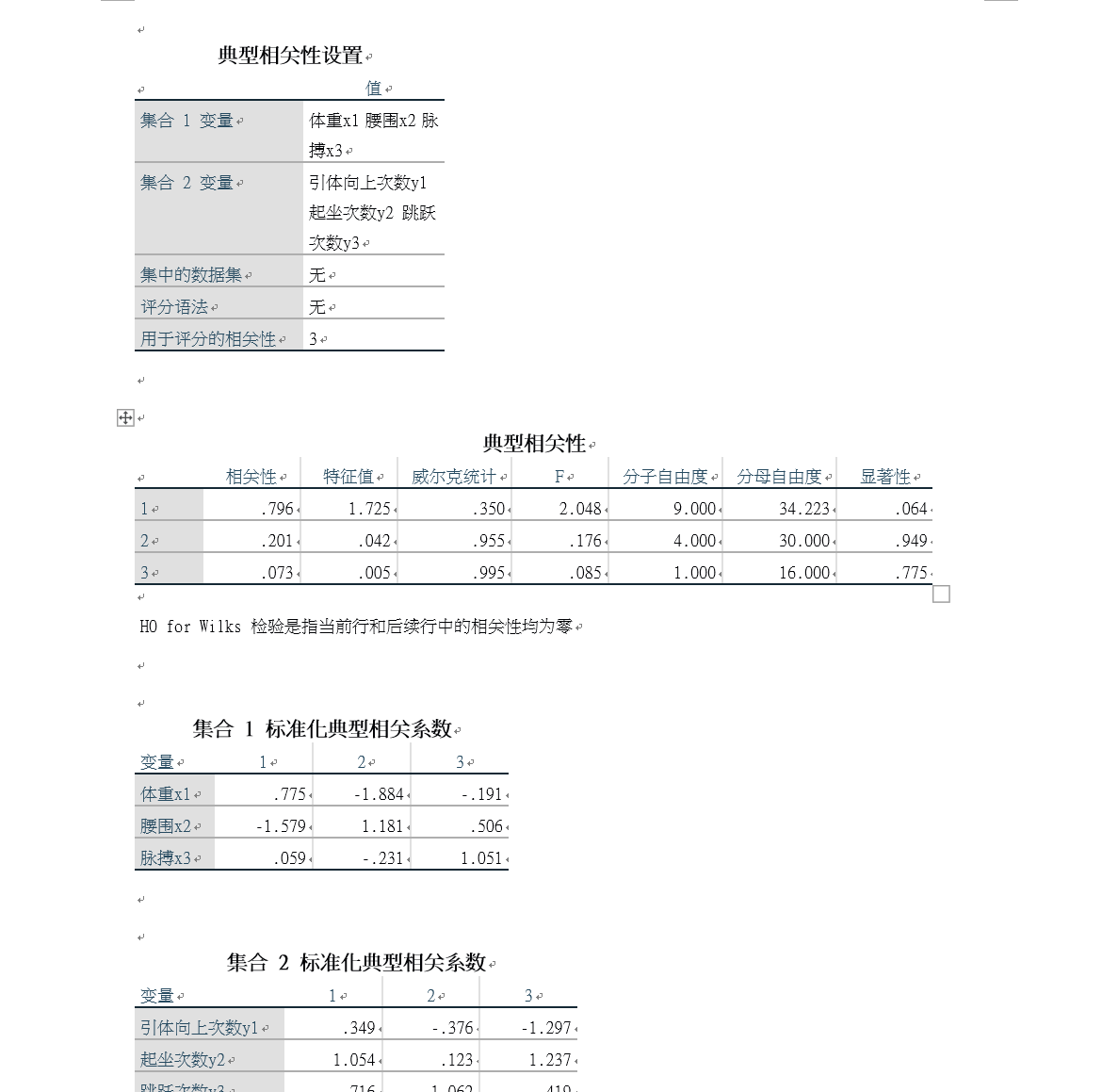将文件中的表格进行重新命名，以免在后续的操作造成干扰。
将所有的集合1修改成生理指标，集合2修改成训练指标。修改表格名称：典型相关性 >>> 典型相关系数修改表格内容：相关性 >>> 相关系数；显著性 >>> p值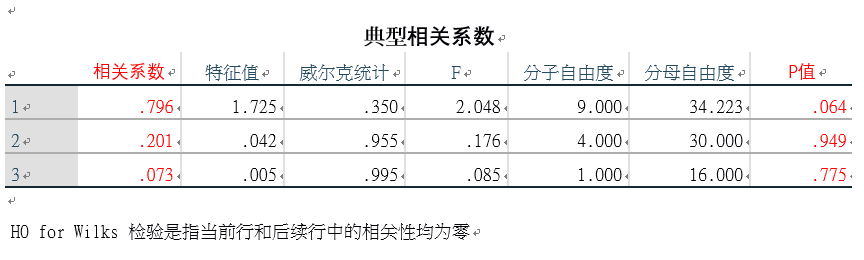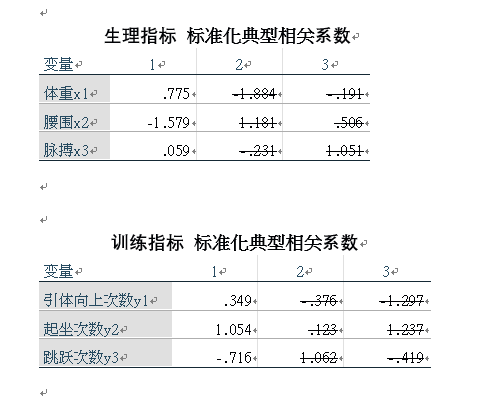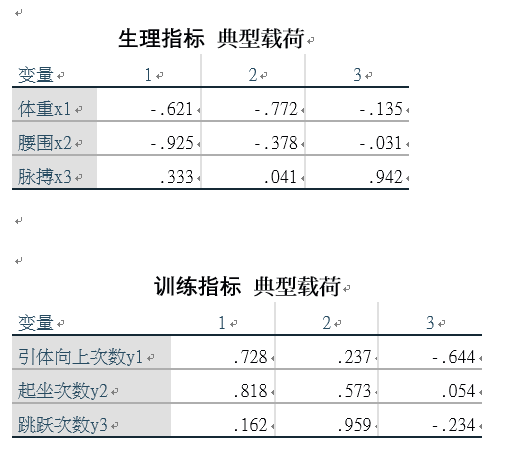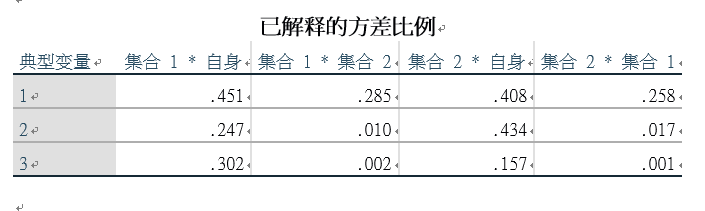注：以上图片，便是我们在建模中经常使用的表格。
五、对结果进行分析
1.分析典型相关系数表该表格的最后一列代表着检验统计量所对应的p值，我们需要通过它确定典型相关系数的个数。我们知道置信水平有三个：90%、95%、99%，其对应的显著性水平分别为 0.1、0.05、0.01.观察第一行的p值，我们发现 0.05 < 0.064 < 0.1. 因此，我们知道在95%的置信水平下，生理指标与训练指标之间不存在相关性；而在90%的置信水平下，生理指标与训练指标之间存在相关性，且第一对典型变量相关性显著。我们接着观察后面两个p值：0.949和0.775。说明第二对和第三对典型变量相关性不显著。由此我们可以确定典型相关系数的个数为1，即第一对典型变量的相关系数。
2. 分析标准化典型相关系数
在该分析中，我们需要写出标准化的典型变量，其个数要根据上一个分析结果所得到的典型相关系数的个数来确定。  在上一个分析结果中我们知道，我们知道我们只需要第一对典型变量的相关系数，因此我们可以将第二、三对的典型变量的相关系数删除。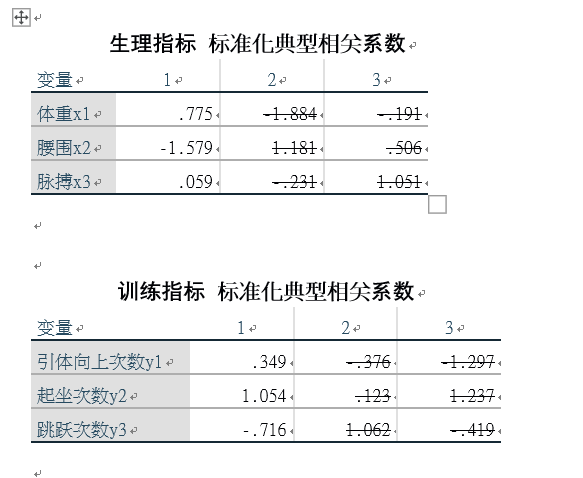由此，可得到的标准化的第一对典型变量：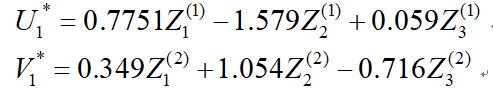其中， Zi(1)和Zj(2)分别为原始变量Xi和Yj标准化后的结果。  典型变量每个分量前面的系数代表着重要程度，可结合典型相关系数进行分析。  结论：
在生理指标中，由于X2（腰围）的绝对值最大，反映生理指标的典型变量主要由腰围决定；在训练指标中，由于Y2（起坐次数）的绝对值最大，说明训练指标的典型变量主要由起坐次数所决定。同时，由于两个典型变量中腰围和起坐次数的系数是异号的（腰围为负，起坐次数为正），反映腰围和起坐次数的负相关，即腰围越小则起坐次数越多。这和客观事实是相符的。
3. 分析典型载荷
说明：为了节省篇幅，在这里笔者只分析生理指标的典型载荷，读者可以模仿分析训练指标的典型载荷。
分析典型载荷的目的：进行典型载荷分析有助于更好解释分析已提取的p对典型变量。所谓的典型载荷分析是指原始变量与典型变量之间相关性分析。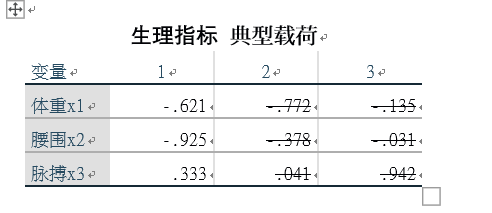分析结果 以上结果说明生理指标的第一典型变量与体重的相关系数为-0.621，与腰围的相关系数为-0.925，与脉搏的相关系数为0.333. 从另一方面说明生理指标的第一对典型变量与体重、腰围负相关，而与脉搏正相关。其中与腰围的相关性最强。生理指标的第一对典型变量主要反映了体型的胖瘦。
4. 分析已解释的方差比例
分析目的 在进行样本典型相关分析时，我们也想了解每组变量提取出的典型变量所能解释的该组样本总方差的比例，从而定量测度典型变量所包含的原始信息量的大小。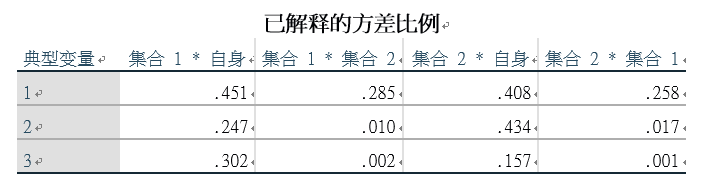数据说明（从左到右）
生理指标被自身的典型变量解释的方差比例；生理指标被训练指标的典型变量解释的方差比例；训练指标被自身的典型变量解释的方差比例；训练指标被生理指标的典型变量解释的方差比例。 分析结果
生理指标样本方差由自身3个典型变量解释的方差比例分别为：
第一典型变量解释的方差比例：0.451；第二典型变量解释的方差比例：0.246，第三典型变量解释的方差比例：0.302； 训练指标样本方差由自身3个典型变量解释的方差比例分别为：
第一典型变量解释的方差比例：0.408；第二典型变量解释的方差比例：0.434；第三典型变量解释的方差比例：0.157；
六、资料链接
资料内容：health.xlsx 链接：https://pan.baidu.com/s/1r3JujIEG3PCfc-K5WskAag 提取码：3exf
展开全文数学建模
• 基于因子分析和典型相关分析的医院发展的三维空间模型，阳长征，何志川，本文首先分析医院由传统式发展向现代化发展过程中存在的问题和面临的挑战，针对这些现状提出了一种医院发展的三维空间模式，即医
• 典型相关分析 摘要 利用典型相关分析的思想 , 提出了解决了当两组特征矢量构成的 总体协方差矩阵奇异时 , 典型投影矢量集的求解问题 , 使之适合于高 维小样本的情形 , 推广了典型相关分析的适用范围 . 首先 , 探讨了...
• 典型相关分析研究与应用，李雪，林和平，经典典型相关分析方法在解决时间序列问题时存在不足：不能及时、准确地反映样本数据的时间特征及变化趋势。针对上述问题，本文基
• 张量典型相关分析_杨慧军.pdf张量典型相关分析_杨慧军.pdf张量典型相关分析_杨慧军.pdf张量典型相关分析_杨慧军.pdf张量典型相关分析_杨慧军.pdfCCA
• 一、什么是典型相关分析 通常情况下，为了研究两组变量 {X=(x1,x2,⋯ ,xp)Y=(y1,y2,⋯ ,yq) \left\{ \begin{array}{l} X=\left( x_1,x_2,\cdots ,x_p \right)\\ \\ Y=\left( y_1,y_2,\cdots ,y_q \right)\\ \...
一、什么是典型相关分析
通常情况下，为了研究两组变量

{

X

=

(

x

1

,

x

2

,

⋯

,

x

p

)

Y

=

(

y

1

,

y

2

,

⋯

,

y

q

)

\left\{ \begin{array}{l} X=\left( x_1,x_2,\cdots ,x_p \right)\\ \\ Y=\left( y_1,y_2,\cdots ,y_q \right)\\ \end{array} \right.

的相关关系，可以用最原始的方法，分别计算两组变量之间的全部相关系数，一共有

p

×

q

p\times q

个简单相关系数，这样又烦琐又不能抓住问题的本质。如果能够采用类似于主成分的思想，分别找出两组变量的各自的某个线性组合，讨论线性组合之间的相关关系，则更简捷。
因此，典型相关分析是分析两组变量之间的相关性的一种统计方法，它包含了简单的Pearson相关分析（两组均只含一个变量）和复相关（一组只含一个变量，另一个组含多个变量）这两种特殊情况。
典型相关分析的基本思想和主成分分析的基本思想相似，它将一组变量与另一组变量之间单变量的多重线性相关性研究，转换为少数几对综合变量之间的简单线性相关性的研究，并且这少数几对变量所包含的线性相关性的信息几乎覆盖了原变量组所包含的全部相应信息。
二、典型相关分析的思想
假设所研究的两组变量为X组和Y组，其中X组有p个变量

X

=

(

x

1

,

x

2

,

⋯

,

x

p

)

X=\left( x_1,x_2,\cdots ,x_p \right)

Y组有q个变量

Y

=

(

y

1

,

y

2

,

⋯

,

y

q

)

\begin{array}{l} Y=\left( y_1,y_2,\cdots ,y_q \right)\\ \end{array}

则分别对这两组变量做线性组合后，再计算它们的加权和的简单相关系数，以这个简单相关系数当做这两组变量之间相关性的度量指标，即

{

u

=

a

1

x

1

+

a

2

x

2

+

⋯

+

a

p

x

p

v

=

b

1

y

1

+

b

2

y

2

+

⋯

+

b

q

y

q

\left\{ \begin{array}{l} u=a_1x_1+a_2x_2+\cdots +a_px_p\\ \\ v=b_1y_1+b_2y_2+\cdots +b_qy_q\\ \end{array} \right.

其中，u 和 v 分别是由 x 变量和 y 变量的线性组合产生的综合逐步变量。显然，对任意的一组系数

(

a

1

,

a

2

,

⋯

,

a

p

)

,

(

b

1

,

b

2

,

⋯

,

b

q

)

\left( a_1,a_2,\cdots ,a_p \right) \text{,}\left( b_1,b_2,\cdots ,b_q \right)

，都可以通过上式求出一对典型变量 u 和 v，在典型相关分析中称之为典型变量。进而可以求出典型变量 u 和 v 的简单相关系数，称之为典型相关系数。
那么，问题来了，怎么进行组合呢？

首先，分别在每组变量中找出第一对线性组合

{

u

1

=

a

11

x

1

+

a

21

x

2

+

⋯

+

a

p

1

x

p

v

1

=

b

11

y

1

+

b

21

y

2

+

⋯

+

b

q

1

y

q

\left\{ \begin{array}{l} u_1=a_{11}x_1+a_{21}x_2+\cdots +a_{p1}x_p\\ \\ v_1=b_{11}y_1+b_{21}y_2+\cdots +b_{q1}y_q\\ \end{array} \right.

使其具有最大相关性，即使得对应的典型变量

u

1

u_1

和

v

1

v_1

的相关系数为最大。假设这个最大的相关系数是

p

1

=

p

(

u

1

,

v

1

)

p_1=p\left( u_1,v_1 \right)

则称

p

1

p_1

为第1典型相关系数，且称具有最大相关系数的这对典型变量

u

1

u_1

和

v

1

v_1

为第1典型变量。
然后再次估计组合系数，在每组变量中找出第二对线性组合，使其分别与本组内的第一线性组合不相关，第二对本身具有次大的相关性

{

u

2

=

a

12

x

1

+

a

22

x

2

+

⋯

+

a

p

2

x

p

v

2

=

b

12

y

1

+

b

22

y

2

+

⋯

+

b

q

2

y

q

\left\{ \begin{array}{l} u_2=a_{12}x_1+a_{22}x_2+\cdots +a_{p2}x_p\\ \\ v_2=b_{12}y_1+b_{22}y_2+\cdots +b_{q2}y_q\\ \end{array} \right.

假设这个次大的相关系数是

p

2

=

p

(

u

2

,

v

2

)

p_2=p\left( u_2,v_2 \right)

则称

p

2

p_2

为第2典型相关系数，且称这对典型变量

u

2

u_2

和

v

2

v_2

为第2典型变量。
其中，

u

2

u_2

和

v

2

v_2

与

u

1

u_1

和

v

1

v_1

相互独立，但

u

2

u_2

和

v

2

v_2

相关。如此继续下去，直至进行到 r 步，两组变量的相关性被提取完为止。

r

≤

m

i

n

(

p

,

q

)

r\le min\left( p,q \right)

可以得到 r 组变量。
从上述分析的过程可以看出，第1对典型变量的第1典型相关系数

p

1

p_1

描述了两个组中变量之间的相关程度，且它提取的有关这两组变量相关性的信息景最多。
第2对典型变量的第2典型相关系数

p

2

p_2

也描述了两个组中变量之间的相关程度，但它提取的有关这两组变量相关性的信总量次多。
依次类推，可以得知，由上述方法得到的一系列典型变量的典型相关系数，所包含的有关原变量组之间相关程度的信息一个比一个少，如果少数几对典型变量就能够解释原数据的主要信息，特别是如果一对典型变量就能够反映出原数据的主要信息，那么，对两个变量组之间相关程度的分析就可以转化为对少数几对或者是一对典型变量的简单相关分析，这就是典型相关分析的主要目的。
三、典型相关分析的理论以及基本假设
考虑两组变量的向量

{

x

=

(

x

1

,

x

2

,

⋯

,

x

p

)

T

y

=

(

y

1

,

y

2

,

⋯

,

y

q

)

T

\left\{ \begin{array}{l} x=\left( x_1,x_2,\cdots ,x_p \right) ^T\\ \\ y=\left( y_1,y_2,\cdots ,y_q \right) ^T\\ \end{array} \right.

典型相关分析就是寻找 x 组 的线性组合

u

1

=

a

11

x

1

+

a

21

x

2

+

⋯

+

a

p

1

x

p

u_1=a_{11}x_1+a_{21}x_2+\cdots +a_{p1}x_p

与 y 组的线性组合

v

1

=

b

11

y

1

+

b

21

y

2

+

⋯

+

b

q

1

y

q

v_1=b_{11}y_1+b_{21}y_2+\cdots +b_{q1}y_q

，使得

u

1

u_1

和

v

1

v_1

之间的简单相关系数为最大，其中

a

1

=

{

a

11

a

12

⋮

a

1

p

b

1

=

{

b

11

b

12

⋮

b

1

q

a_1=\left\{ \begin{array}{l} \begin{array}{c} a_{11}\\ a_{12}\\ \end{array}\\ \begin{array}{c} \vdots\\ a_{1p}\\ \end{array}\\ \end{array} \right. \ \ \ \ \ \ \ b_1=\left\{ \begin{array}{l} \begin{array}{c} b_{11}\\ b_{12}\\ \end{array}\\ \begin{array}{c} \vdots\\ b_{1q}\\ \end{array}\\ \end{array} \right.

设，x 组与 y 组的协方差阵为

Σ

=

[

Σ

x

x

Σ

x

y

Σ

y

x

Σ

y

y

]

\Sigma =\left[ \begin{matrix} \varSigma _{xx}& \varSigma _{xy}\\ \varSigma _{yx}& \varSigma _{yy}\\ \end{matrix} \right]

其中

Σ

x

x

\varSigma _{xx}

是 x 组 的协方差矩阵；

Σ

y

y

\varSigma _{yy}

是 y 组变量的协方差矩阵；

Σ

x

y

=

Σ

y

x

′

\Sigma _{xy}=\Sigma '_{yx}

是 x 组和 y 组 的协方差矩阵。
则

u

1

u_1

的方差为

V

a

r

(

u

1

)

=

V

a

r

(

a

1

′

x

)

=

a

1

′

Σ

x

x

a

1

Var\left( u_1 \right) =Var\left( a_1'x \right) =a_1'\varSigma _{xx}a_1

v

1

v_1

的方差为

V

a

r

(

v

1

)

=

V

a

r

(

b

1

′

y

)

=

b

1

′

Σ

y

y

b

1

Var\left( v_1 \right) =Var\left( b_1'y \right) =b_1'\varSigma _{yy}b_1

u

1

u_1

和

v

1

v_1

的协方差为

C

o

v

(

u

1

,

v

1

)

=

C

o

v

(

a

1

′

x

,

b

1

′

y

)

=

a

1

′

Σ

x

y

b

1

Cov\left( u_1,v_1 \right) =Cov\left( a_1'x,b_1'y \right) =a_1'\varSigma _{xy}b_1

u

1

u_1

和

v

1

v_1

的简单相关系数（pearson）为

p

(

u

1

,

v

1

)

=

C

o

v

(

u

1

,

v

1

)

V

a

r

(

u

1

)

×

V

a

r

(

v

1

)

=

a

1

′

Σ

x

y

b

1

a

1

′

Σ

x

x

a

1

b

1

′

Σ

y

y

b

1

p\left( u_1,v_1 \right) =\frac{Cov\left( u_1,v_1 \right)}{\sqrt{Var\left( u_1 \right) \times Var\left( v_1 \right)}}=\frac{a_1'\varSigma _{xy}b_1}{\sqrt{a_1'\varSigma _{xx}a_1b_1'\varSigma _{yy}b_1}}

典型相关问题就是使得

u

1

u_1

和

v

1

v_1

的简单相关系数最大，故： 在

V

a

r

(

u

1

)

=

1

Var\left( u_1 \right) =1

和

V

a

r

(

v

1

)

=

1

Var\left( v_1 \right)=1

的条件下，求取使

C

o

v

(

u

1

,

v

1

)

Cov\left( u_1,v_1 \right)

最大的

a

1

a_1

和

b

1

b_1

。
然后再次估计组合系数，直至进行到 r 步，两组变量的相关性被提取完为止。

r

≤

m

i

n

(

p

,

q

)

r\le min\left( p,q \right)

可以得到 r 组变量。求出每一组特征值，满足

λ

1

≥

λ

2

≥

⋯

≥

λ

r

\lambda _1\ge \lambda _2\ge \cdots \ge \lambda _r

a

i

a_i

和

b

i

b_i

分别是

λ

i

\lambda _i

的特征向量，则

u

i

=

a

i

′

x

u_i=a_i'x

和

v

i

=

b

i

′

y

v_i=b_i'y

，称为第 i 组典型变量，

u

i

u_i

和

v

i

v_i

的第 i 典型相关为

λ

i

，

i

=

1

,

2

,

⋯

,

r

\sqrt{\lambda _i}\text{，}i=1,2,\cdots ,r

因为，

Σ

x

x

,

Σ

y

y

,

Σ

x

y

\varSigma _{xx},\varSigma _{yy},\varSigma _{xy}

未知，改以

S

x

x

,

S

y

y

,

S

x

y

S_{xx},S_{yy},S_{xy}

取代之，而得到

λ

^

1

≥

λ

^

2

≥

⋯

≥

λ

^

r

\hat{\lambda}_1\ge \hat{\lambda}_2\ge \cdots \ge \hat{\lambda}_r

典型变量的系数称为典型权重，权重越大，表示此变量对此典型变量的贡献越大。在以上的计算中，此权重为标准后的资源所得的，故 k 个资料的第 i 典型变量得点为

∑

a

i

j

(

x

j

k

−

x

ˉ

j

)

s

j

\sum_{}{a_{ij}\frac{\left( x_{jk}-\bar{x}_j \right)}{s_j}}

四、冗余分析
冗余分析是通过原始变量与典型变量之间的相关性。分析引起原始变量变异的原因。以原始变量为因变量，以典型变量为自变量，建立线性回归模型，则相应的确定系数（判定系数

R

2

R^2

）等于因变量与典型变量间的相关系数的平方，它描述了由于因变量与典型变量的线性关系引起的因变量变异在因变量的总变异中比例。
典型负荷为变量与典型变量的相关系数，可由相关系数的平方了解此典型变量解释了此变量多少比例的变异数。
五、典型相关系数的假设检验
那么，要选择多少组典型变量呢？
在做两组变量 X，Y 的典型相关分析之前，首先应该检验两组变量是否相关，如果不相关，则讨论两组变量的典型相关就毫无意义.
最多可以选取 r = min(p,q) 组，可经由卡方检验决定要选取多少组典型变量。先检验最大的典型根，然后再一个接一个对各个根进行检验，只保留有统计显著性（就是拒绝原假设）的根。
（1）提出假设

H

0

：

λ

1

=

λ

2

=

λ

3

=

⋯

=

λ

r

=

0

↔

H

1

：

λ

1

,

λ

2

,

λ

3

,

⋯

,

λ

r

至少有一个不为

0

H_0\text{：}\lambda _1=\lambda _2=\lambda _3=\cdots =\lambda _r=0\leftrightarrow H_1\text{：}\lambda _1,\lambda _2,\lambda _3,\cdots ,\lambda _r\text{至少有一个不为}0

检验统计量

χ

2

=

−

[

(

n

−

1

)

−

p

+

q

+

1

2

]

ln

⁡

(

1

−

λ

^

1

)

(

1

−

λ

^

2

)

(

1

−

λ

^

3

)

⋯

(

1

−

λ

^

r

)

\chi ^2=-\left[ \left( n-1 \right) -\frac{p+q+1}{2} \right] \ln \left( 1-\hat{\lambda}_1 \right) \left( 1-\hat{\lambda}_2 \right) \left( 1-\hat{\lambda}_3 \right) \cdots \left( 1-\hat{\lambda}_r \right)

其中， r = min(p,q)，若

χ

2

>

χ

α

2

(

p

q

)

\chi ^2>\chi _{\alpha}^2\left( pq \right)

，则拒绝原假设，则认为至少第一对典型变量

u

1

u_1

和

v

1

v_1

具有相关性，得出第一对典型变量

u

1

u_1

和

v

1

v_1

可能已经提取了两组变量X 和 Y相关关系的绝大部分信息，

λ

1

\lambda _1

是显著的，是具有价值的，那么，接下来将它移除，再检验剩余 r -1 组的显著性，否则接受原假设，认为两组变量之间的相关性没有意义。
（2）当上述原假设

H

0

H_0

被拒绝时，接着做

H

0

：

λ

2

=

λ

3

=

⋯

=

λ

r

=

0

H_0\text{：}\lambda _2=\lambda _3=\cdots =\lambda _r=0

检验统计量

χ

2

=

−

[

(

n

−

1

)

−

p

+

q

+

1

2

]

ln

⁡

(

1

−

λ

^

2

)

(

1

−

λ

^

3

)

⋯

(

1

−

λ

^

r

)

\chi ^2=-\left[ \left( n-1 \right) -\frac{p+q+1}{2} \right] \ln \left( 1-\hat{\lambda}_2 \right) \left( 1-\hat{\lambda}_3 \right) \cdots \left( 1-\hat{\lambda}_r \right)

其中， r = min(p,q)，若

χ

2

>

χ

α

2

(

(

p

−

1

)

(

q

−

1

)

)

\chi ^2>\chi _{\alpha}^{2}\left( \left( p-1 \right) \left( q-1 \right) \right)

则拒绝原假设，则认为第二对典型变量

u

2

u_2

和

v

2

v_2

具有相关性，认为至少前两组具有相关性，可能已经提取大部分信息，

λ

2

\lambda _2

也是显著的，是具有价值的，那么，接下来将它移除，再检验剩余 r -2 组的显著性。
（3）当上述原假设

H

0

H_0

被拒绝时，接着做

H

0

：

λ

3

=

⋯

=

λ

r

=

0

H_0\text{：}\lambda _3=\cdots =\lambda _r=0

…
（4）依次类推，直到在检验某个 t 值 ，没有理由拒绝原假设为止，即认为至少前t-1组典型变量是相关的，都是具有价值的，可能已经提取 X 和 Y 相关关系的绝大部分信息，没有必要对剩余的组进行检验了。

H

0

：

λ

t

=

⋯

=

λ

r

=

0

H_0\text{：}\lambda _t=\cdots =\lambda _r=0

事实上，检验的总显著性水平已不是α了，且难以确定。还有，检验的结果易受样本容量大小的影响，因此，检验的结果只宜作为确定典型变量个数的重要参考依据，而不宜作为惟一的依据。
关于典型相关分析的具体操作
实际上，只需要掌握典型相关分析的基本原理，基本思想就可以了，具体实际操作并不难，不需要通过公式进行计算，目前基本上是使用SPSS进行分析。 这里推荐两个教程： https://mp.weixin.qq.com/s/cI0wXAjRIjQkPC_xAfbQpw
https://mp.weixin.qq.com/s/cQiDk4ZsKvvcl9E5kaakIg
展开全文...